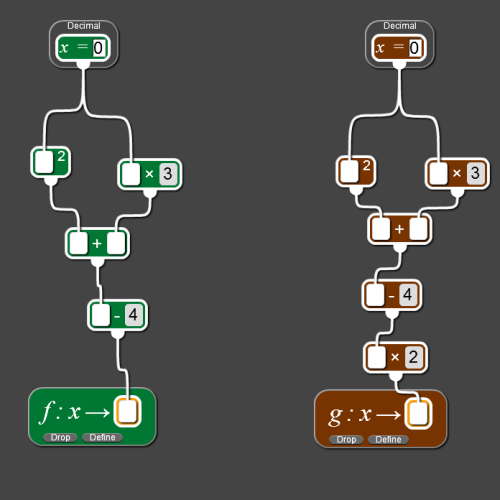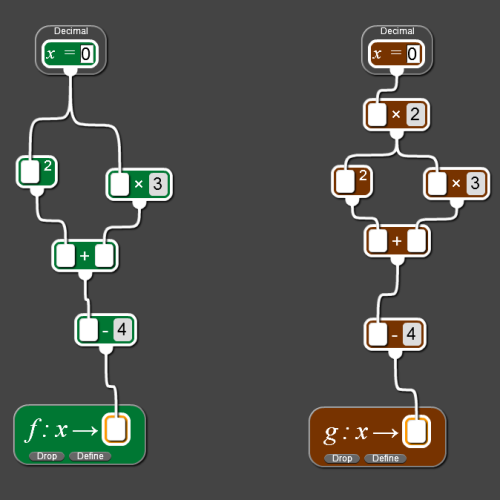You may also likeConverse

Clearly if a, b and c are the lengths of the sides of an equilateral triangle then a^2 + b^2 + c^2 = ab + bc + ca. Is the converse true?Consecutive Squares

The squares of any 8 consecutive numbers can be arranged into two sets of four numbers with the same sum. True of false?Parabolic Patterns

The illustration shows the graphs of fifteen functions. Two of them have equations y=x^2 and y=-(x-4)^2. Find the equations of all the other graphs.

Age 14 to 16 Challenge Level:
If you have never used the NRICH Number Plumber before, click here to watch a short introductory video.

Click on the image below to investigate the two functions and their graphs.Try using the same input number for both functions. What do you notice?
Look at the steps which make up the functions.
How are the two functions related?
How are their graphs related?

If you have met the idea of expressing a function in the form f(x) , try to write one function in terms of the other.

Experiment with other pairs of functions linked in the same way.

Click on the image below to investigate two more functions and their graphs.Try to find pairs of input numbers so that both machines give the same output. What do you notice?
Look at the steps which make up the functions.
How are the two functions related?
How are their graphs related?

If you have met the idea of expressing a function in the form f(x), try to write one function in terms of the other.

Experiment with other pairs of functions linked in the same way.